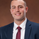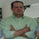TradingView
Dilihat 3312
The Herrick Payoff Index is designed to show the amount of money flowing into or out of a futures contract. The Index uses open interest during its calculations, therefore, the security being analyzed must contain open interest.

The Herrick Payoff Index was developed by John Herrick.When the Herrick Payoff Index is above zero, it shows that money is flowing into the futures contract (which is bullish ). When the Index is below zero, it shows that money is flowing out of the futures contract (which is bearish ).

The interpretation of the Herrick Payoff Index involves looking for divergences between the Index and prices.
Skrip open-source

Sejalan dengan semangat TradingView, penulis skrip ini telah mempublikasikannya secara open-source, sehingga para trader dapat memahami dan memverifikasinya. Salut untuk sang penulis! Anda dapat menggunakannya secara gratis, tetapi penggunaan kembali kode ini dalam publikasi diatur oleh Tata Tertib. Anda dapat memfavoritkannya untuk dapat menggunakannya didalam sebuah chart.

Inggin menggunakan skrip ini pada chart?
```////////////////////////////////////////////////////////////
//  Copyright by shardison v1.0 08/05/2015
// Credit to GREENY for his work on the COT OPEN INTEREST SCRIPT
// Attention:
//The Herrick Payoff Index (HPI) was developed by John Herrick and is the only well known indicator that uses price, volume, and open interest.
// The Herrick Payoff Index is designed to show the amount of money flowing into or out of a futures contract.
//The Index uses open interest during its calculations, therefore, the security being analyzed must contain open interest.
//NOTE: THE COT OPEN INTEREST SCRIPT ONLY pulls Weekly DATA, hence only utilize this script on Weekly bars.
//Interpretation
//
//When the Herrick Payoff Index is above zero, it shows that money is flowing into the futures contract (which is bullish).
//When the Index is below zero, it shows that money is flowing out of the futures contract (which is bearish).
//
//The interpretation of the Herrick Payoff Index involves looking for divergences between the Index and prices.
////////////////////////////////////////////////////////////

study(title="Herrick Payoff Index", shorttitle="HPI")
valueofonecentmove = input(100, minval=1)
multiplyingfactor = input(10, minval=1)
wmaperiod = input(21, minval=1)
force_root = input("", title="Override Product")
is_includeoptions = input(false, type=bool, title="Include Options")

fxroot =
ticker == "USDCAD" ? "CD" :
ticker == "USDCAD" ? "CD" :
ticker == "USDCHF" ? "SF" :
ticker == "USDCZK" ? "CZ" :
ticker == "USDHUF" ? "FR" :
ticker == "USDILS" ? "IS" :
ticker == "USDJPY" ? "JY" :
ticker == "USDMXN" ? "MP" :
ticker == "USDNOK" ? "UN" :
ticker == "USDPLN" ? "PZ" :
ticker == "USDRUB" ? "RU" :
ticker == "USDSEK" ? "SE" :
ticker == "USDZAR" ? "RA" :
ticker == "EURUSD" ? "EC" :
ticker == "AUDUSD" ? "AD" :
ticker == "GBPUSD" ? "BP" :
ticker == "NZDUSD" ? "NE" :
ticker == "BRLUSD" ? "BR" :
""

is_inversed =
ticker == "USDCAD" ? true :
ticker == "USDCAD" ? true :
ticker == "USDCHF" ? true :
ticker == "USDCZK" ? true :
ticker == "USDHUF" ? true :
ticker == "USDILS" ? true :
ticker == "USDJPY" ? true :
ticker == "USDMXN" ? true :
ticker == "USDNOK" ? true :
ticker == "USDPLN" ? true :
ticker == "USDRUB" ? true :
ticker == "USDSEK" ? true :
ticker == "USDZAR" ? true :
false

root = force_root == "" ? fxroot == "" ? syminfo.root : fxroot : force_root
code = root + (is_includeoptions ? "_FO" : "_F") + "_L_ALL"

oi = security("QUANDL:CFTC/"+code+"|0", "D", close)

commercial_long_total = security("QUANDL:CFTC/"+code+"|4", "W", close)
commercial_short_total = security("QUANDL:CFTC/"+code+"|5", "W", close)
long_total = security("QUANDL:CFTC/"+code+"|1", "W", close)
short_total = security("QUANDL:CFTC/"+code+"|2", "W", close)

commercial_long = is_inversed ? commercial_short_total : commercial_long_total
commercial_short = is_inversed ? commercial_long_total : commercial_short_total
long = is_inversed ? short_total : long_total
short = is_inversed ? long_total : short_total

diff = commercial_long - commercial_short
cdiff = diff - diff
cI = abs(cdiff)
cG = max(diff, diff)
diff2 = long - short
openinterestdiff = oi - oi
I = abs(openinterestdiff)
G = max(oi, oi)
S = multiplyingfactor
C = valueofonecentmove
V = volume
M = (high + low) / 2
My = M
K1 = (C*V*(M - My))*(1 + ((2 * I)/(G)))
K2 = (C*V*(M - My))*(1 - ((2 * I)/(G)))
K = M > My ? K1 : K2
Ky = K
HPI = ((Ky +(K - Ky)) * S)/100000
HPI_Index = 100 * (HPI - lowest(HPI,100))/(highest(HPI,100) - lowest(HPI,100))
wma = wma(HPI, wmaperiod)
plot(HPI, color=green, title="HPI")
plot(HPI_Index, color=aqua, title="HPI Index-Turn off all others")
plot (wma, color=orange, title="HPI Weighted Moving Average")
plot (diff, color=red, title="Commercials Net-Position")
plot (diff2, color=blue, title="Non-Commercials Net-Position")
hline(0, color=gray, title="Zero", linestyle=dashed)
//plot(oi, color = gray, title="OI")```

## KomentarWell done on this! Thank you for adding this to the community!
Jawabringarg12
@ringarg12, This specific indicator only works for futures contracts, because it uses the CFTC data to calculate open interest. So you would need to use something like CLK2018 or alike for crude oil instead of USOIL.
Jawab
Profil Pengaturan Profil Akun dan Penagihan Teman yang di refer Koin Tiket Dukungan Saya Pusat Bantuan Pesan Pribadi Obrolan Keluar